• ## 递归算法时间复杂度分析

万次阅读 多人点赞 2018-09-17 16:16:59
递归算法时间复杂度分析 时间复杂度： 一般情况下，算法中基本操作重复的次数就是问题规模n的某个函数f（n），进而分析f（n）随n的变化情况并确定T（n）的数量级。这里用‘o’来表示数量级，给出算法时间复杂度。 T...
                             递归算法时间复杂度分析

时间复杂度：

一般情况下，算法中基本操作重复的次数就是问题规模n的某个函数f（n），进而分析f（n）随n的变化情况并确定T（n）的数量级。这里用‘o’来表示数量级，给出算法时间复杂度。
T（n）=o（f（n））；
它表示随问题规模n的增大，算法的执行时间增长率和f（n）增长率成正比，这称作算法的渐进时间复杂度。而我们一般情况下讨论的最坏的时间复杂度。
空间复杂度：
算法的空间复杂度并不是实际占用的空间，而是计算整个算法空间辅助空间单元的个数，与问题的规模没有关系。算法的空间复杂度S（n）定义为该算法所耗费空间的数量级。
S（n）=o（f（n））
若算法执行所需要的辅助空间相对于输入数据n而言是一个常数，则称这个算法空间复杂度辅助空间为o（1）；
递归算法空间复杂度：递归深度n*每次递归所要的辅助空间，如果每次递归所需要的辅助空间为常数，则递归空间复杂度o（n）。

递归算法分析

1、利用数列知识

累加法：递推关系式为an+1−an=f(n)an+1−an=f(n)采用累加法。
累乘法：递推关系式为an+1an=f(n)an+1an=f(n)采用累乘法。
构造法：递推关系式为(1)aa+1=pan+qaa+1=pan+q，(2)aa+1=pan+qnaa+1=pan+qn，都可以通过恒等变形，构造出等差或等比数列，利用等差或等比数列的定义进行解题，其中的构造方法可通过待定系数法来进行。
和化项法：递推公式为Sn=f(n)Sn=f(n)或Sn=f(an)Sn=f(an)一般利用
an={S1，Sn−Sn−1，当n=1当n>=2an={S1，当n=1Sn−Sn−1，当n>=2

用特征方程求解递推方程（感觉比较生僻，不做解释）
迭代法： 从原始递推方程开始，反复将对于递推方程左边的函数用右边的等式代入，直到得到初值，然后将所得的结果进行化简。
例如在调用归并排序mergeSort(a,0,n-1)对数组a[0...n−1]a[0...n−1]排序时，执行时间T(n)T(n)的递推关系式为：
T(n)={O(1)，2T(n2)+O(n)，当n=1当n>=2T(n)={O(1)，当n=12T(n2)+O(n)，当n>=2

其中，O(n)O(n)为merge()所需要的时间，设为cncn（c为正常量）。因此：
T(n)=2T(n2)+cn=2(2T(n4)+cn2)+cn=22T(n4)+2cn=23T(n8)+3cn=...=2kT(n2k)+kcn=nO(1)+cnlog2n=O(nlog2n),(假设n=2k,则k=log2n)T(n)=2T(n2)+cn=2(2T(n4)+cn2)+cn=22T(n4)+2cn=23T(n8)+3cn=...=2kT(n2k)+kcn=nO(1)+cnlog2⁡n=O(nlog2⁡n),(假设n=2k,则k=log2⁡n)

忽略求解细节。在我们求解递归式时，因为最终是要求得一个时间上限，所以在求解时常常省略一些细节。比如mergeSort(a,0,n-1)运行时间的实际递归式应该是：

T(n)={O(1)，T(⌈n2⌉)+T(⌊n2⌋)+O(n)，当n=1当n>=2T(n)={O(1)，当n=1T(⌈n2⌉)+T(⌊n2⌋)+O(n)，当n>=2

但我们忽略这些上取整、下取整以及边界条件，甚至假设问题规模n=2kn=2k，这都是为方便求解而忽略的细节。经验和一些定理告诉我们，这些细节不会影响算法时间复杂度的渐近界。

类似的，我们也可以用迭代法求解汉诺塔递归求解时的时间复杂度。但遗憾的是，迭代法一般适用于一阶的递推方程。对于二阶及以上（即T(n)依赖它前面更多个递归项T(n)依赖它前面更多个递归项）的递推方程，迭代法将导致迭代后的项太多，从而使得求和公式过于复杂，因此需要将递推方程化简，利用差消法等技巧将高阶递推方程化为一阶递推方程。如在求快速排序算法平均时间复杂度T(n)T(n)的递推方程，T(n)T(n)依赖T(n−1)、T(n−2)、...、T(1)T(n−1)、T(n−2)、...、T(1)等所有的项，这样的递推方程也称为全部历史递推方程。（这里省略快速排序算法平均复杂度T(n)的求解过程）

小结：上面6种递推关系是高中、本科知识，在此重点介绍了迭代法，其它几种方法虽未在本篇中使用，但可以加深对递推式求解的认识。

2、代入法

代入法实质上就是数学归纳法，因此求递推式分为两步：

猜测解的形式；
用数学归纳法求出解中的常数，并证明解是正确的。
遗憾的是并不存在通用的方法来猜测递归式的正确解，需要凭借经验，偶尔还需要创造力。即使猜出了递归式解的渐近界，也有可能在数学归纳证明时莫名其妙的失败。正是由于该方法技术细节较为难掌握，因此这个方法不适合用来求解递归方程，反而比较适合作为其他方法检验手段。在此不做总结。可以翻阅《算法导论》进行学习。

3、递归树

递归树是一棵结点带权值的树。初始的递归树只有一个结点，它的权标记为T(n)T(n)；然后按照递归树的迭代规则不断进行迭代，每迭代一次递归树就增加一层，直到树中不再含有权值为函数的结点（即叶结点都为T(1)T(1)）。下面以递归方程

T(n)={O(1)，2T(n2)+O(n)，当n=1当n>=2;(假设n=2k,则k=log2n)T(n)={O(1)，当n=12T(n2)+O(n)，当n>=2;(假设n=2k,则k=log2⁡n)

来讲述递归树的迭代规则。

第一步： 把根结点T(n)T(n)用根是cncn、左结点为T(n2)T(n2)、右结点为T(n2)T(n2)的子树代替（即：以分解、合并子问题需要的代价为根，分解得到的子问题为叶的子树。其中常量c代表求解规模为1的问题所需的时间）；（如下如(a)→(b)(a)→(b)）
第二步：把叶结点按照“第一步”的方式展开；T(n2)T(n2)用根是cn/2cn/2、左节点为T(n4)T(n4)、右结点为T(n4)T(n4)的子树代替。（如下如(b)→(c)(b)→(c)）
第三步：反复按照“第一步”的方式迭代，每迭代一次递归树就增加一层，直到树中不再含有权值为函数的结点（即叶结点都为T(1)T(1)）。（如下如(c)→(d)(c)→(d)）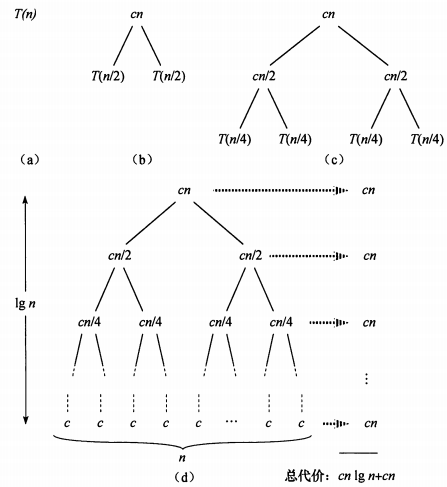在得到递归树后，将树中每层中的代价求和，得到每层代价，然后将所有层的代价求和，得到所有层次的递归调用的总代价。在上图(d)部分中，完全展开的递归树高度为lgnlg⁡n(树高为根结点到叶结点最长简单路径上边的数目)，所有递归树具有lgn+1lg⁡n+1层，所以总代价为cn∗(lgn+1)cn∗(lg⁡n+1)，所有时间复杂度为Θ(nlgn)Θ(nlg⁡n)。

总结：递归树模型求解递归方程，本质上就是迭代思想的应用，利用递归方程迭代展开过程构造对应的递归树，然后把每层的时间代价进行求和。不过递归树模型更直观，同时递归树也克服了二阶及更高阶递推方程不方便迭代展开的痛点。

4、主方法求解递推式

主方法为如下形式的递归式提供了一种“菜谱”式的求解方法，如下所示

T(n)=aT(n/b)+f(n)T(n)=aT(n/b)+f(n)

其中a≥1a≥1和b>1b>1是常数，f(n)f(n)是渐近正函数。这个递推式将规模为n的问题分解为a个子问题，每个子问题的规模为n/bn/b，a个子问题递归地求解，每个花费时间T(n/b)T(n/b)。函数f(n)f(n)包含了问题分解和子问题解合并的代价。同样，这个递归式也没有考虑上取整、下取整、边界条件等，结果不会影响递归式的渐近性质。

定理4.1(主定理) 令a≥1和b>1是常数，f(n)f(n)是一个函数，T(n)T(n)是定义在非负整数上的递归式：

T(n)=aT(n/b)+f(n)T(n)=aT(n/b)+f(n)

其中我们将n/bn/b解释为⌊n/b⌋⌊n/b⌋或⌈n/b⌉⌈n/b⌉。那么T(n)T(n)有如下渐近界：
1. 若对某个常数ε>0ε>0有f(n)=O(n(logba)−ε)f(n)=O(n(logb⁡a)−ε)，则T(n)=Θ(nlogba)T(n)=Θ(nlogb⁡a)
2. 若f(n)=Θ(nlogba)f(n)=Θ(nlogb⁡a)，则T(n)=Θ(nlogbalgn)T(n)=Θ(nlogb⁡alg⁡n)。
3. 若对某个常数ε>0ε>0有f(n)=Ω(n(logba)+ε)f(n)=Ω(n(logb⁡a)+ε)，且对某个常数c<1c<1和所有足够大的n有af(n/b)≤cf(n)af(n/b)≤cf(n)，则T(n)=Θ(f(n))T(n)=Θ(f(n))

在使用主定理之前，要比较f(n)和(nlogba)f(n)和(nlogb⁡a)的大小，这个大小不是算术意义上的大小比较，而是要在多项式意义上比较。以上三种情况在多项式意义上并未覆盖f(n)f(n)的所有可能性。情况1和情况2之间有一定间隙；情况2和情况3之间也有一定间隙。如果f(n)落在这两个间隙中，或者情况3中 正则条件不成立，就不能使用主方法来求递归式。
如在递归式：T(n)=2T(n/2)+nlgnT(n)=2T(n/2)+nlg⁡n中，因为 nlogba=n<f(n)=nlgnnlogb⁡a=n<f(n)=nlg⁡n，但是f(n)f(n)并不大于n一个多项式因子nεnε ,因为对于给定的ε>0ε>0当n足够大时，均有nε>lgnnε>lg⁡n。所以找不到这样 ε>0ε>0，该递归式落入了情况2和情况3之间的间隙，不能使用主定理。
最后给出主定理应用的几个练习题：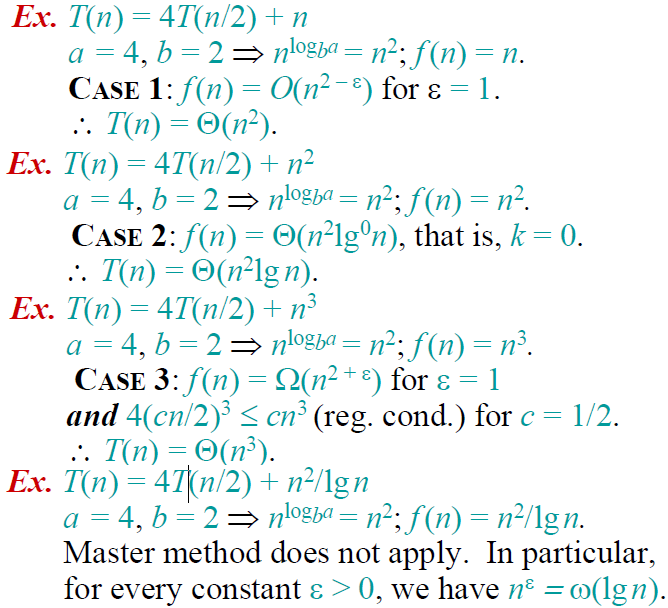具体举例分析：

【代入法】代入法首先要对这个问题的时间复杂度做出预测，然后将预测带入原来的递归方程，如果没有出现矛盾，则是可能的解，最后用数学归纳法证明。

【举   例】我们有如下的递归问题：T(n)=4T(n/2)+O(n)，我们首先预测时间复杂度为O(n2),不妨设T(n)=kn2（其中k为常数），将该结果带入方程中可得：左=kn2，右=4k(n/2)2+O(n)=kn2+O(n),由于n2的阶高于n的阶，因而左右两边是相等的，接下来用数学归纳法进行验证即可。

【迭代法】迭代法就是迭代的展开方程的右边，直到没有可以迭代的项为止，这时通过对右边的和进行估算来估计方程的解。比较适用于分治问题的求解，为方便讨论起见，给出其递归方程的一般形式：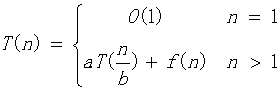【举   例】下面我们以一个简单的例子来说明：T(n)=2T(n/2)+n2,迭代过程如下：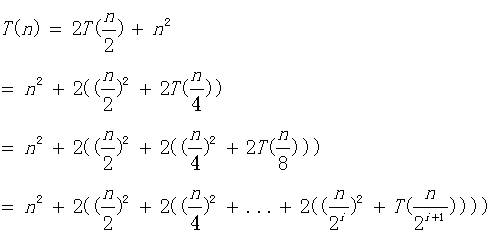容易知道，直到n/2^(i+1)=1时，递归过程结束，这时我们计算如下：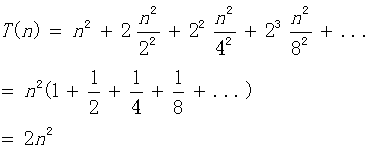到这里我们知道该算法的时间复杂度为O(n2)，上面的计算中，我们可以直接使用无穷等比数列的公式，不用考虑项数i的约束，实际上这两种方法计算的结果是完全等价的，有兴趣的同学可以自行验证。

【公式法】这个方法针对形如：T(n) = aT(n/b) + f(n)的递归方程。这种递归方程是分治法的时间复杂性所满足的递归关系，即一个规模为n的问题被分成规模均为n/b的a个子问题，递归地求解这a个子问题，然后通过对这a个子问题的解的综合，得到原问题的解。这种方法是对于分治问题最好的解法，我们先给出如下的公式：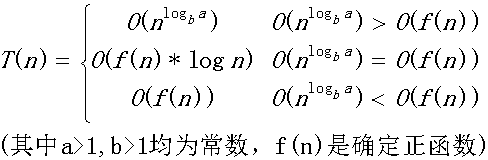公式记忆：我们实际上是比较n^logba和f(n)的阶，如果他们不等，那么T(n)取他们中的较大者，如果他们的阶相等，那么我们就将他们的任意一个乘以logn就可以了。按照这个公式，我们可以计算【迭代法】中提到的例子：O(f(n))=O(n2),容易计算另外一个的阶是O(n),他们不等，所以取较大的阶O(n2)。太简单了，不是吗？

需要注意：上面的公式并不包含所有的情况，比如第一种和第二种情况之间并不包含下面这种情况：f(n)是小于前者，但是并不是多项式的小于前者。同样后两种的情况也并不包含所有的情况。为了好理解与运用的情况下，笔者将公式表述成如上的情况，但是并不是很严谨，关于该公式的严密讨论，请看这里。但是公式的不包含的情况，我们很少很少碰到，上面的公式适用范围很广泛的。

特别地，对于我们经常碰到的，当f(n)=0时，我们有：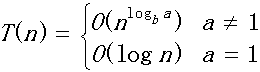【母函数法】母函数是用于对应于一个无穷序列的幂级数。这里我们解决的递归问题是形如：T(n)=c1T(n-1)+c2T(n-2)+c3T(n-3)+...+ckT(n-k)+f(n)。为说明问题简便起见，我们选择斐波那契数列的时间复杂度作为例子进行讨论。

【举  例】斐波那契数列递归公式：T(n)=T(n-1)+T(n-2)。这里我们假设F(n)为第n项的运算量。则容易得到：F(n)=F(n-1)+F(n-2),其中F(1)=F(2)=1.我们构造如下的母函数：G(x)=F(1)x+F(2)x2+F(3)x3+......,我们可以推导如下：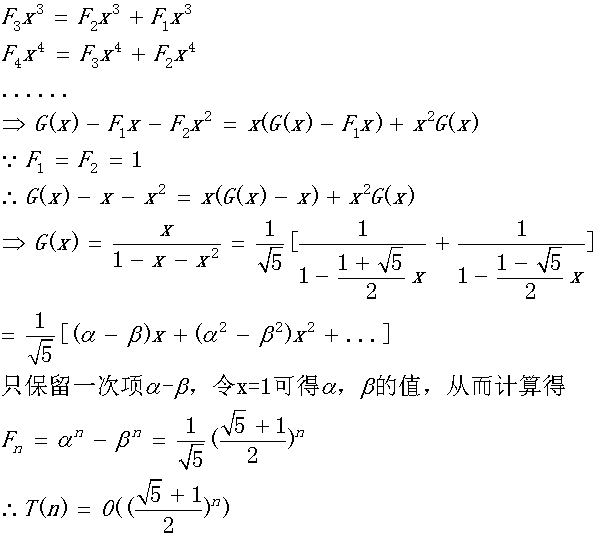上面的方法计算相对来说是比较简单的，关键在于对于母函数的理解，刚开始的时候可能不是很好理解，对于母函数可以参考这里和维基百科这里。

【差分方程法】可以将某些递归方程看成差分方程，通过解差分方程的方法来解递归方程，然后对解作出渐近阶估计。这里我们只考虑最长常见的递归形式，形如：T(n)=c1T(n-1)+c2T(n-2)+c3T(n-3)+...+ckT(n-k)+f(n)，其中c1,c2,...ck为常数且不等于0；我们对该方程的求解如下：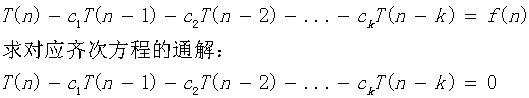对应上面的齐次方程的特征方程为：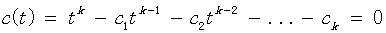如果解得t=r是该特征方程的m重根，则这m个解的形式为：{rn     n*rn      n2rn   ...    nm-1rn},其余的关于复数解的形式和普通的线性方程组的形式类似，不再赘述。接下来，我们要求解该方程的对应非齐次方程组的通解，这里我们针对该方程的特殊形式，不加证明地给出如下的通解形式：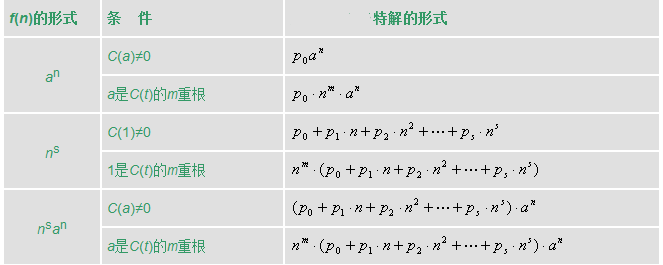则和线性代数中的解一样，原方程的解等于齐次方程组的通解+特解，即：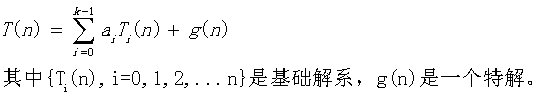最后由初始条件确定a(i)的值即可。

为了帮助理解，我们举两个例子看看，就明白是怎么回事了。

【举 例1】递归方程如下：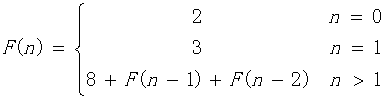(1)写出对应齐次方程的特征方程：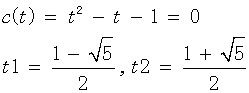得到基础解系为：{t1n,  t2n}

(2)计算特解，对于本题，直接观察得特解为：-8

(3)得到原方程解的形式为：T(n)=a0t1n+a1t2n-8

(4)代入n=0，n=1的情况，得到a0,a1，最后可得: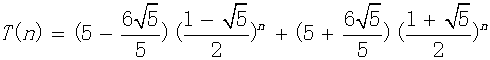可以看到该方程形式和上面讨论过的斐波那契数列只差一个常数8，因而两者的时间复杂度是相同的。有兴趣的同学可以按照这个方法再次计算斐波那契数列的时间复杂度验证一下。

【举  例2】递归方程如下：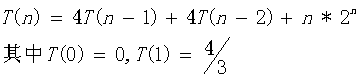（1）计算对应齐次方程的基础解析：特征方程为：C(t)=t^2-4t-4=0,得到一个2重根t=2.因而其基础解为：{2n      n*2n}

（2）由于f(n)=n*2n，对应上面表格的最后一种情况，得到特解形式为：T(n)=n2(p0+p1n)2n代入原递归方程可得：p0=1/2,p1=1/6

（3）原方程解的形式为：T(n)=a0*2n+a1*n*2n+n2(1/2+n/6)2n,代入T(0),T(1)得：a0=a1=0

（4）综上：T(n)=n2(1/2+n/6)2n

因而时间复杂度为：O(n32n).

参考：https://blog.csdn.net/so_geili/article/details/53444816

https://blog.csdn.net/m0_37734999/article/details/78751882

https://www.cnblogs.com/hlongch/p/5749038.html

展开全文• 算法时间复杂度分析 在看一个算法是否优秀时，我们一般都要考虑一个算法时间复杂度和空间复杂度。现在随着空间越来越大，时间复杂度成了一个算法的重要指标，那么如何估计一个算法时间复杂度呢？ 时间复杂度...
算法时间复杂度分析

在看一个算法是否优秀时，我们一般都要考虑一个算法的时间复杂度和空间复杂度。现在随着空间越来越大，时间复杂度成了一个算法的重要指标，那么如何估计一个算法的时间复杂度呢？
时间复杂度直观体现
首先看一个时间复杂度不同的两个算法，解决同一个问题，会有多大的区别。
下面两个算法都是用来计算斐波那契数列的，两个算法会有多大的差异。

斐波那契数列（Fibonacci sequence），又称黄金分割数列、因数学家列昂纳多·斐波那契（Leonardoda Fibonacci）以兔子繁殖为例子而引入，故又称为“兔子数列”，指的是这样一个数列：1、1、2、3、5、8、13、21、34、……在数学上，斐波那契数列以如下被以递推的方法定义：F(1)=1，F(2)=1, F(n)=F(n-1)+F(n-2)（n>=3，n∈N*）

第一种：使用递归方式

    /**
* 使用递归方式计算斐波拉契数列
* @param  index 计算的项数
*/
public static long fibonacciUseRecursion(int index){
if(index <= 1){
return index;
}
return fibonacciUseRecursion(index-1) + fibonacciUseRecursion(index-2);
}


第二种：使用非递归方式

    /**
* 不使用递归方式计算斐波拉契数列
* @param index 计算的项数
*/
public static long fibonacciNoUseRecursion(int index){
if (index <= 1){
return index;
}
long first = 0;
long second = 1;
for (int i = 0; i < index - 1;i++){
second = first + second;
first = second - first;
}
return second;
}

对上面两种算法进行简单的运行时间统计，我们使用下面的代码进行简单的测试
public static void main(String[] args) {
// 获取当前时间
long begin = System.currentTimeMillis();
// 计算第50项斐波拉契数列的值
System.out.println(fibonacciUseRecursion(50));
// 计算时间差，算法执行所花的时间
System.out.println("time:" + (System.currentTimeMillis() - begin) / 1000 +"s");

begin = System.currentTimeMillis();
System.out.println(fibonacciNoUseRecursion(50));
System.out.println("time:" + (System.currentTimeMillis() - begin) / 1000 + "s");
}

测试结果如下: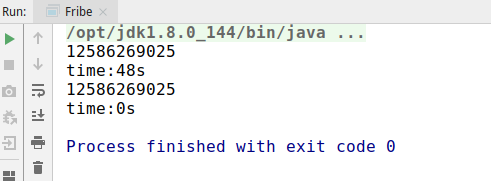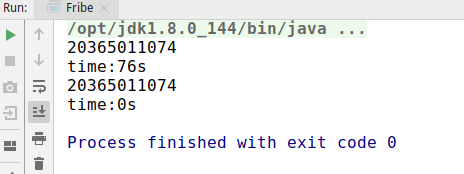可以看到，在计算第50项的时候，第一种递归方式花费了48秒的时间，而第二种不到一秒，虽然这种方式不太科学，但也看出来了两者巨大的差距，并且随着计算的值越大，时间的差异越明显。由此可见，时间复杂度是决定一个算法好坏的重要指标。
如何衡量一个算法的好坏

正确性、可读性、健壮性。
算法必须要保证正确，不正确的算法是没有必要衡量其好坏的；算法也要保证良好的可读性，能够让阅读者明白内在实现与逻辑；健壮性为对不合理输入的反应能力和处理能力，比如非法输入，要有相应的处理，而不应该程序奔溃等。这些都是一个良好的算法必备的条件。
时间复杂度
时间复杂度也是一个衡量算法优劣的重要条件，不同的算法的执行时间可能会存在很大的差别。
空间复杂度
空间复杂度表示一个算法执行过程中，需要的空间(内存)数量，也是衡量一个算法的重要指标，尤其是在嵌入式等程序中的算法，内存是非常宝贵的，有时候宁愿提高时间复杂度，也要保证不占用太多的空间。

如何计算时间复杂度
第一种：事后统计法
上面我们使用了一种计算执行前后时间差的方式，直观的来看一个算法的复杂度，比较不同算法对同一组输入的执行时间，这种方法也叫作"事后统计法"，但是这种方法也存在一些问题，主要问题有：

执行时间严重依赖于硬件已经运行时各种不确定的环境因素。
比如两个算法在不同的硬件机器上进行测试，硬件不同，运行时间也会存在差异，即使就在一台机器上执行，也会存在运行时机器的CPU、内存使用情况不同等因素。
必须要编写相应的测试代码。
测试数据的选择难以保证公正性。
比如有两个算法，一个在数据量小的时候占优，一个在大数据量的时候运行较快，这样便难以选择一个公正的测试数据。

第二种：估算代码指令执行次数
那么我们可以使用代码的每个指令的执行次数，可以简单估算代码的执行次数，一般情况下，执行次数少的肯定要比执行次数多的花的时间更少。看如下的示例：
    public static void test1(int n) {
if (n > 10) {
System.out.println("n > 10");
} else if (n > 5) {
System.out.println("n > 5");
} else {
System.out.println("n <= 5");
}

for (int i = 0; i < 4; i++) {
System.out.println("test");
}
}

上面这个方法，我们计算它的执行次数。

最上面的if…else if…else这个判断，判断会执行一次、判断成立的代码会执行一次。
下面的for循环，i=0这句赋值会执行一次，i<4这个判断条件会执行4次，i++也会执行4次，循环体(输出语句)也会执行4次。
因此，整个方法的执行次数为：1+1+1+4+4+4 = 15次。

    public static void test2(int n) {
for (int i = 0; i < n; i++) {
System.out.println("test");
}
}

上面这个方法，我们计算它的执行次数。

在for循环中，i=0这句赋值会执行一次，i < n执行n次，i++执行n次，循环体执行n次。
因此，整个方法的执行次数为：1+n+n+n = 3n+1 次

    public static void test3(int n) {
for (int i = 0; i < n; i++) {
for (int j = 0; j < n; j++) {
System.out.println("test");
}
}
}

上面这个方法，我们计算它的执行次数。

在外层for循环中，i=0这句赋值会执行一次，i < n执行n次，i++执行n次，循环体执行n次。
在内层循环中，j=0这句赋值会执行一次，j < n执行n次，j++执行n次，循环体执行n次。
因此，整个方法的执行次数为 1+n+n+n*(1+n+n+n)=3n2+3n+1 次

    public static void test4(int n) {
for (int i = 0; i < n; i++) {
for (int j = 0; j < 15; j++) {
System.out.println("test");
}
}
}


上面这个方法，我们计算它的执行次数。

在外层for循环中，i=0这句赋值会执行一次，i < n执行n次，i++执行n次，循环体执行n次。
在内层循环中，j=0这句赋值会执行一次，j < 15执行15次，j++执行15次，循环体执行15次。
因此，整个方法的执行次数为 1+n+n+n*(1+15+15+15)=48n+1 次

    public static void test5(int n) {
while ((n = n / 2) > 0) {
System.out.println("test");
}
}

上面这个方法，我们计算它的执行次数。

在while循环中，每次对n取一半，相当于对n取以二为底的对数，因此n = n / 2 会执行log2(n)次，判断条件也会执行log2(n)次。
在循环体中，这个输出语句也会执行log2(n)次。
因此，整个方法的执行次数为 log2(n) + log2(n) + log2(n) = 3log2(n)次

    public static void test6(int n) {
while ((n = n / 5) > 0) {
System.out.println("test");
}
}

上面这个方法，我们计算它的执行次数。

在while循环中，每次对n取五分之一，相当于对n取以五为底的对数，因此n = n / 5 会执行log5(n)次，判断条件也会执行log5(n)次。
在循环体中，这个输出语句也会执行log5(n)次。
因此，整个方法的执行次数为 log5(n) + log5(n) + log5(n) = 3log5(n)次

    public static void test7(int n) {
for (int i = 1; i < n; i = i * 2) {
for (int j = 0; j < n; j++) {
System.out.println("test");
}
}
}

上面这个方法，我们计算它的执行次数。

在外层for循环中，i= 1执行一遍，每次i翻倍，执行次数为log2(n)，因此i < n会执行log2(n)次，i=i*2会执行log2(n)次，循环体执行log2(n)。
在内层for循环中，j=0执行一次，j < n执行n次，j++执行n次，内层循环条件执行n次。
因此，整个方法的执行次数为 1+ log2(n) + log2(n) + log2(n)*(1+n+n+n) = 3nlog2(n) + 3log2(n)+1次

    public static void test8(int n) {
int a = 10;
int b = 20;
int c = a + b;
int[] array = new int[n];
for (int i = 0; i < array.length; i++) {
System.out.println(array[i] + c);
}
}

上面这个方法，我们计算它的执行次数。

a=10执行一次，b=20执行一次，c=a+b执行一次，初始化数组执行一次。
在for循环中，i=0执行一次，i < 数组长度执行n次，i++执行n次，内层循环条件执行n次。
因此，整个方法的执行次数为 1+1+1+1+1+n+n+n =3n +5次。

使用这种方法我们发现计算会特别麻烦，而且不同的时间复杂度表达书也比较复杂，我们也不好比较两个时间复杂度的具体优劣，因此为了更简单、更好的比较不同算法的时间复杂度优劣，提出了一种新的时间
复杂度表示法—大O表示法。
大O表示法

大O表示法：算法的时间复杂度通常用大O符号表述，定义为T[n] = O(f(n))。称函数T(n)以f(n)为界或者称T(n)受限于f(n)。 如果一个问题的规模是n，解这一问题的某一算法所需要的时间为T(n)。T(n)称为这一算法的“时间复杂度”。当输入量n逐渐加大时，时间复杂度的极限情形称为算法的“渐近时间复杂度”。

大O表示法，用来描述复杂度，它表示的是数据规模n对应的复杂度，大O表示法有以下的一些特性：

忽略表达式常数、系数、低阶项。
忽略常数，常数直接为1，比如上面第一个方法的复杂度为15，因此直接取1，其时间复杂度使用大O表示为O(1)。
忽略系数，忽略表达式的系数，比如第二个方法的时间复杂度为3n+1，忽略系数和常数，其时间复杂度为O(n)。
忽略低阶项，比如第三个方法的时间复杂度为3n2+3n+1,忽略低阶项3n，忽略常数1，忽略系数3，则其时间复杂度为O(n2)。
对数阶一般忽略底数
对于对数直接的转换，一个对数都可以乘以一个常数项成为一个没有底数的对数，比如
log2n = log29 * log9n，因此可以省略底数，比如上面第五个方法的时间复杂度为log2(n),可以忽略底数2，则其时间负责度为logn。
大O表示法仅仅是一种粗略的分析模型，是一种估算，能帮助我们短时间内估算一个算法的时间复杂度。

常见的复杂度

执行次数
复杂度
非正式术语

12
O(1)
常数阶

2n+3
O(n)
线性阶

4n2+zn+2
O(n2)
平方阶

4log2n+21
O(logn)
对数阶

3n+2log3n+15
O(nlogn)
nlogn阶

4n3+3n2+22n+11
O(n3)
立方阶

2n
O(2n)
指数阶

复杂度的大小关系
O(1) < O(logn) < O(n) < O(nlogn) < O(n2) < O(n3) < O(2n) < O(n!) < O(nn)。
因此上面的十个方法的复杂度如下：

方法名称
复杂度
大O表式

test1
15
O(1)

test2
3n+1
O(n)

test3
3n2+3n+1
O(n2)

test4
48n+1
O(n)

test5
3log2(n)
O(logn)

test6
3log5(n)
O(logn)

test7
3nlog2(n) + 3log2(n) + 1
O(nlogn)

test8
3n+5
O(n)

直观对比复杂的的大小
直接看表达式，还是很难判断一些复杂度的大小关系，我们可以借助可视化的一些工具来查看比如https://zh.numberempire.com/graphingcalculator.php，通过该网站我们看到在n变化的情况下，不同表达式的变换情况。
递归斐波拉契数列计算方法的时间复杂度分析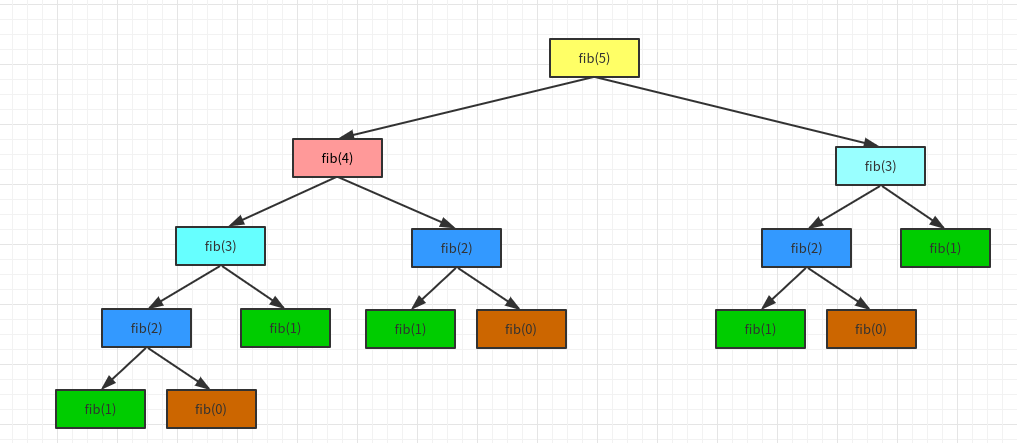第一层计算5，只需要计算1次；第二层计算3和4，2次；计算第3层，4次；计算第4层，8次。所以总共计算1+2+4+8 =15= 25-1 = 1/2 * 22 -1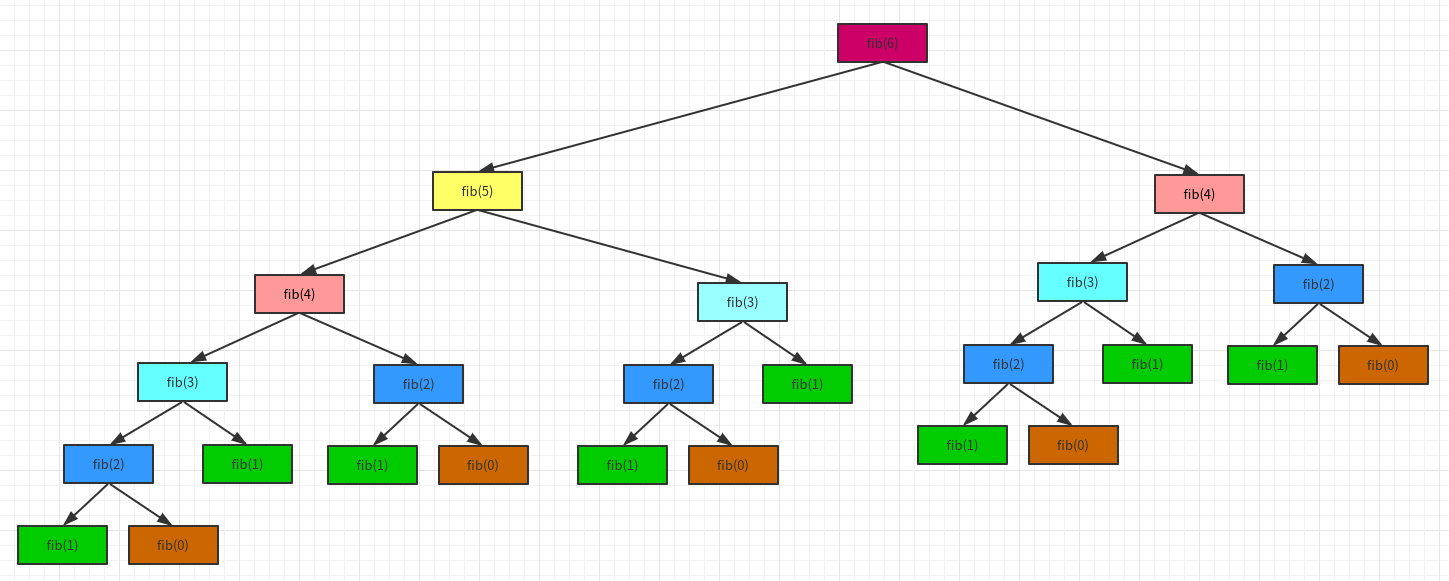第一层计算6，只需要计算1次；第二层计算5和4，2次；计算第3层，4次；计算第4层，8次；第5层，计算10次。所以总共计算1+2+4+8+10 =25 = 25 - 7 = 1/2 * 26 - 7。
所以计算第n项，它的时间复杂度为O(2^n)。
所以最开始的两个算法，第一个的算法复杂度为O(2n),一个为O(n)。
他们的差别有多大？

如果有一台1GHz的普通计算机，运算速度109次每秒（n为64）
O(n)大约耗时6.4 ∗ 10-8秒
O(2n)大约耗时584.94年
有时候算法之间的差距，往往比硬件方面的差距还要大

算法的优化方向

用尽量少的存储空间，即空间复杂度低。
用尽量少的执行步骤，即时间复杂度低。
一定情况下，时间复杂度和空间复杂度可以互换。

关于复杂度的更多概念

最好、最坏复杂度
均摊复杂度
复杂度震荡
平均复杂度
…

总结展开全文java
• 汉诺塔与斐波那契数的算法时间复杂度分析 一.汉诺塔 部分代码 void Hanoi(int n, char a, char b, char c)//a为原始柱，b为借助柱,c为目标柱 { if (n == 1) { Move(a, c);//只有一个盘子时直接移 } else { ...
汉诺塔与斐波那契数的算法时间复杂度分析
一.汉诺塔
部分代码
void Hanoi(int n, char a, char b, char c)//a为原始柱，b为借助柱,c为目标柱
{
if (n == 1)
{
Move(a, c);//只有一个盘子时直接移
}
else
{
Hanoi(n - 1, a, c, b);//将A柱子上n-1个盘子借助C柱子移到B上
Move(a, c);//将A最后一个盘子移到C上
Hanoi(n - 1, b, a, c);//将B柱子借助空A柱子移到C上
}
}

void Move(char orin, char target)
{
cout << orin << "->" << target << endl;
}

算法分析
设盘子个数为n时，需要T（n)步，把A柱子n-1个盘子移到B柱子，需要T（n-1)步，A柱子最后一个盘子移到C柱子一步，B柱子上n-1个盘子移到C柱子上T（n-1)步。
得递推公式T（n）=2T（n-1)+1
T (n)以及O (n)推导如下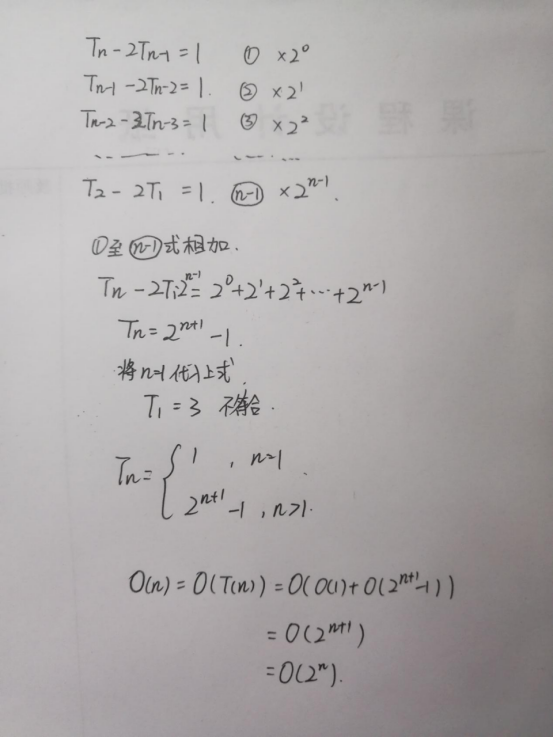二.斐波那契数列
部分代码
long long Fbnum(long long N)
{
if (N < 3)    //当N<3时，斐波那契数为1
return 1;
else
return Fbnum(N - 1) + Fib(N - 2);//函数递归
}
int main()
{
int n = 0;
scanf("%d",&n);
printf("%d",Fbnum(n));
return 0;
}

算法分析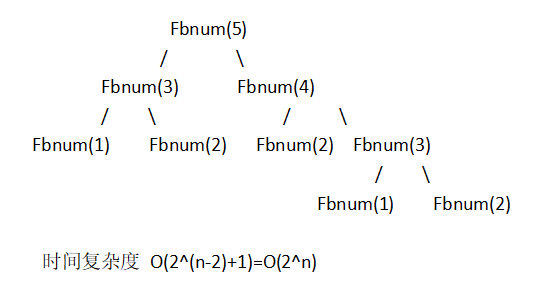展开全文汉诺塔
• 正式工作也有3年的时间了，想要写出更加优雅的代码。所以最近在刷leetcode补充数据结构和算法方面的知识。学校里虽然学过，但是仅仅是有个大概的认识。... 有两个衡量优劣的维度：时间复杂度和空...正式工作也有3年的时间了，想要写出更加优雅的代码。所以最近在刷leetcode补充数据结构和算法方面的知识。学校里虽然学过，但是仅仅是有个大概的认识。只有实际工作过几年以后，才会明白数据结构和算法的重要性。如果是通信专业出身的同学，或者是硬件出身的同学一定知道：对于一个信号，我们可以从时域和频域两个方面去分析。那么计算机科学或者说软件开发中的算法怎么去分析呢？ 有两个衡量优劣的维度：时间复杂度和空间复杂度。时间复杂度：执行当前算法所消耗的'时间'。空间复杂度：执行当前算法所占用的内存。在这边博文中，我们来好好分析一下时间复杂度。时间复杂度真的是计算'时间'吗？时间复杂度公式：大O符号表示法常见时间复杂度类型及代码分析 常数型O(1)对数型O(log n)线性型O(n)线性对数型O(n log n)平方型O(n^2)、立方型O(n^3)、K次方型O(n^k)平方底指数型O(2^n)、立方底指数型O(3^n)、K次底指数型O(k^n)阶乘型O(n!)如何理解斐波那契数列的时间复杂度O(2^N)?如何理解阶乘型时间复杂度O(n!)？参考资料时间复杂度真的是计算'时间'吗？把算法的执行时间当做时间复杂度？ 这种方式是最为直观也是最容易想到的方式。 但是有一个问题，那就是代码在不同性能的机器上运行，以及在不同的状态下运行，会呈现出完全不同的运行时间。 比如说我有一台内存为32GB内存的mbp，还有一台8GB的台式机，假设其它的硬件条件比如cpu，主板以及机器负载状态一致。通常情况下，32GB的内存要比8GB的内存运行更快。而且这种理想状态下的只有单一变量的状态也是很难做到的。 所以不能通过计算算法的消耗时间作为时间复杂度。那我们通常所说的'时间'复杂度中的'时间'到底是指什么呢？聪明的前辈们想到了一种方式：大O表示法。大O表示法内部有非常复杂的数学计算逻辑，我们偷个懒，不去证明公式，把公式用好就很厉害了。 为什么不去证明一下或者演算一遍？ 我在大一曾经上过一门叫做高等代数的课，有道题目叫做：请证明1+1=2。 看到这个题目应该知道为什么不深究大O表示法背后的数学了吧。时间复杂度公式：大O符号表示法T(n) = O(f(n))
f(n)是指每行代码执行次数之和f(n)可以是这些值：1，log n，n，nlog n，n^2，n^3，n^k，2^n，n!f(n)与O正相关O(f(n))可以是这些值：O(1)，O(log n)，O(n)，O(nlog n)，O(n^2)，O(n^3)，O(n^k)，O(2^n)，O(n!)大O表示法实际表示的是代码执行时间的增长变化趋势，不是真实的运行时间，是一种趋势预估大O表示法中的f(n)是近似值。很多时候不会完全是1，log n，n，nlog n，n^2，n^3，n^k，2^n，n!这些完整的值。例如斐波那契数列的真实时间复杂度为O(2^N-1)，由于N->∞，所以可以近似为O(2^N)。 更多的斐波那契数列时间复杂度的分析可以查看下文中的：如何理解斐波那契数列的时间复杂度O(2^N)?常见时间复杂度类型及代码分析理论扯了一大堆了，到精彩绝伦的Show me the code环节了。 先来看一张大O复杂度曲线图。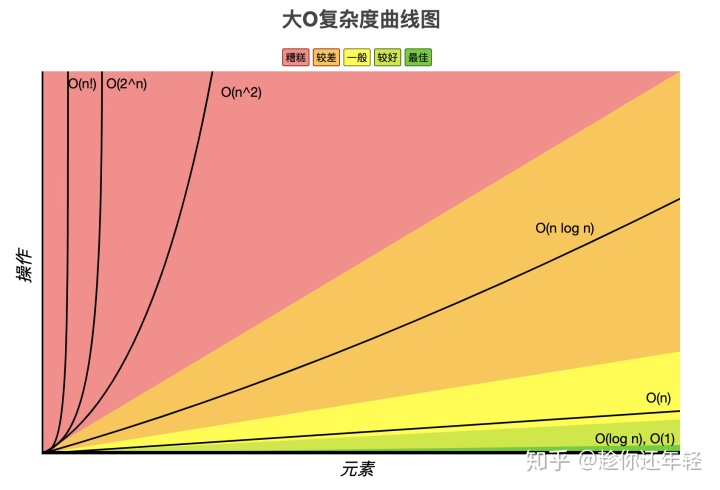以下时间复杂度根据最佳->较好->一般->较差->糟糕的顺序排列。常数型O(1)对数型O(log n)线性型O(n)线性对数型O(n log n)平方型O(n^2)、立方型O(n^3)、K次方型O(n^k)平方底指数型O(2^n)、立方底指数型O(3^n)、K次底指数型O(k^n)阶乘型O(n!)常数型O(1)常见于赋值和引用等简单操作算法消耗不随变量增长而增长，性能最佳无论代码执行多少行，即使有几千几万行，时间复杂度都为O(1)实际开发过程中，一次递归的时间复杂度也为O(1)。因为O(1^n)无论n为多少都为O(1)let i = 0;
let j = 9;
i++;
j--;
let k = i + j;代码分析： i为1，j为10，k为11。 时间复杂度为O(1)。对数型O(log n)常用代码执行次数为x，n为目标数字。符合2^x=n，推导出x=log2(n)（log n）的情况算法消耗随n的增长而增长，性能较好let n = 100;
let i = 1;
while(i<n){
i = i * 2
}代码分析： i为128。 n为100，时间复杂度为O(log2(100))。 因为Math.log2(100)≈6.64，所以最终的时间复杂度为O(6.65)。线性型O(n)常见于一次for循环，while循环算法消耗随n的增长而增长，性能一般无论n值有多大，即使是Inifinity，时间复杂度都为O(n)let n = 100;
let j = 0;
for(let i = 0;i<n;i++){
j = i;
}代码分析： i为100，j为99。 n为100，时间复杂度为O(100)。线性对数型O(n log n)常用于一个对时间复杂度为O(log2(n))的代码执行一个n次循环算法消耗随n的增长而增长，性能较差let n = 100;
for(let m = 0; m<n; m++){
let i = 1;
while(i<n){
i = i * 2
}
}代码分析： i为128。 m为100，n为100，时间复杂度为O(m log2(n))。 因为100* Math.log2(100)≈664.39，所以最终的时间复杂度为O(664.39)。平方型O(n^2)、立方型O(n^3)、K次方型O(n^k)最常见的算法时间复杂度，可用于快速开发业务逻辑常见于2次for循环，或者3次for循环，以及k次for循环算法消耗随n的增长而增长，性能糟糕实际开发过程中，不建议使用K值过大的循环，否则代码将非常难以维护let n = 100
let v = 0;
for(let i =0;i<n;i++){
for(let j = 0; j<n; j++){
v = v+j+i;
}
}代码分析： v为990000，i为100，j为100. n为100，时间复杂度为O(100^2)。 也就是O(10000)。立方型O(n^3)、K次方型O(n^k)和平方型O(n^2)类似，无非是多了几次循环。// 立方型O(n^3)
for(let i =0;i<n;i++){
for(let j = 0; j<n; j++){
for(let m = 0; m<n; m++){

}
}
}
// K次方型O(n^k)
for(let i =0;i<n;i++){
for(let j = 0; j<n; j++){
for(let m = 0; m<n; m++){
for(let p = 0; p<n; p++){
... // for循环继续嵌套下去，k值不断增大
}
}
}
}平方底指数型O(2^n)、立方底指数型O(3^n)、K次底指数型O(k^n)常见于2次递归的情况，3次递归以及k次递归的情况算法消耗随n的增长而增长，性能糟糕实际开发过程中，k为1时，一次递归的时间复杂度为O(1)。因为O(1^n)无论n为多少都为O(1)。斐波那契数列（兔子数列、黄金分割数列）：1、1、2、3、5、8、13、21、34··· 题目：leetcode 509 斐波那契数 题解：509.斐波那契数/**
* @param {number} N
* @return {number}
*/
var fib = function (N) {
/**
* 解法1: 递归
* 性能:  88ms 34.2MB
* 时间复杂度：O(2^N)
*/
if (N <= 1) return N;
return fib(N - 1) + fib(N - 2);
};假设N等于100。 代码分析： 结果为 xxx。 因为浏览器直接卡死。nodejs中也运行不出来。 具体原因则是2的100次方真的太大了。算不来。 N为100，时间复杂度为O(2^100)。 因为Math.pow(2, 100)= 1.2676506002282294e+30，所以最终的时间复杂度为O(1.2676506002282294e+30)。大到爆表。立方底指数型O(3^n)、K次底指数型O(k^n)与平方底指数型O(2^n)类似，只不过基数变为了3和k。O(Math.pow(3, n))
O(Math.pow(k, n))假设n为100，假设k为5。 Math.pow(3, n)为5.153775207320113e+47。 Math.pow(5, n)为7.888609052210118e+69。 时间复杂度也是巨高，真的是指数爆炸 。更多的斐波那契数列时间复杂度O(2^N）的分析可以查看下文中的：如何理解斐波那契数列的时间复杂度O(2^N)?阶乘型O(n!)极其不常见算法消耗随n的增长而增长，性能糟糕function nFacRuntimeFunc(n) {
for(let i=0; i<n; i++) {
nFacRuntimeFunc(n-1);
}
}阶乘型O(n!)的时间复杂度按照(n!+(n-1)!+(n-2)!+ ··· + 1) +((n-1)!+(n-2)!+ ··· + 1)+ ···  的方式去计算。 注意哦，这里是多个阶乘的和。不仅仅是n * (n-1) * (n-2) * (n-3)···1。 假设n从0到10，它的算法复杂度O(n!)依次为1，4，15，64，325，1956，13699，109600，986409，9864100··· 为了和上文中的其它算法复杂度做比较，n为100时是多少呢？ O(2^n)为10才是1024，n为100时O(2^n)直接浏览器卡死了。 O(n!)才为10就接近1000万了，真要是n设置成100，计算到机器烧了也计算不出吧。 所以n为100时的O(n!)就不要想了，庞大到恐怖的一个数字。更多的阶乘型时间复杂度O(n!)的分析可以查看下文中的：如何理解阶乘型算法复杂度O(n!)？如何理解斐波那契数列的时间复杂度O(2^N)?O(2^N)Math.pow(base, ex)，2个递归所以base是2。N的话是因为N->∞，但其实真正是O(2^(N-1))。/**
* @param {number} N
* @return {number}
*/
var fib = function (N) {
/**
* 解法1: 递归
* 性能:  88ms 34.2MB
*/
console.log('foo');
if (N <= 1) return N;
return fib(N - 1) + fib(N - 2)
};N打印foo数O(2^N)11O(2^0)22^1 + 1O(2^1)32^2 + 1O(2^2 )42^3 + 1O(2^3 )52^4 + 1O(2^4 )通过上表我们分析得到： 如果包含1的话，严格来讲时间复杂度是O(2^(N-1))。 如果从N>1开始计算，时间复杂度确实是O(2^N)。 斐波那契数列非常长，N->∞，因此可以将斐波那契数列的时间复杂度直接看做是O(2^N)。如何理解阶乘型时间复杂度O(n!)？O(N!)我们把上面的代码改造一下，增加一个count用来统计O(n!)。let count = 0;
function nFacRuntimeFunc(n) {
for(let i=0; i<n; i++) {
count++;
nFacRuntimeFunc(n-1);
}
}阶乘型O(n!)的时间复杂度按照(n!+(n-1)!+(n-2)!+ ··· + 1) +((n-1)!+(n-2)!+ ··· + 1) 的方式去计算。 注意哦，这里是多个阶乘的和。不仅仅是n * (n-1) * (n-2) * (n-3)···1。 上述示例中的count即为复杂度的值。n多次n! + (n-1)! + ··· + 1!countO(n!)111O(1)2(2!+1!) +(1!)4O(4)3(3!+(2!+1!)+1!)+((2!+1!)+1!)+(1!)15O(15)4...64O(64)5...325O(325)6...1956O(1956)7...13699O(13699)8...109600O(109600)9...986409O(986409)10...9864100O(9864100)快看看这个表格吧，n为10的时候O(n!)达到了O(9864100)，接近了O(一千万)。这种算法的性能真的是糟糕到极致了。参考资料https://juejin.im/post/5e7c0946f265da42e879fe0c https://zhuanlan.zhihu.com/p/50479555 https://www.bigocheatsheet.com/ https://stackoverflow.com/questions/3953244/example-of-on 期待和大家交流，共同进步，欢迎大家加入我创建的与前端开发密切相关的技术讨论小组： 微信公众号： 生活在浏览器里的我们 / excellent_developersGithub博客: 趁你还年轻233的个人博客SegmentFault专栏：趁你还年轻，做个优秀的前端工程师Leetcode讨论微信群：Z2Fva2FpMjAxMDA4MDE=（加我微信拉你进群）努力成为优秀前端工程师！
展开全文• 今天来简单聊聊算法复杂度这个概念，算法复杂度包含时间复杂度和空间复杂度二个方面。 时间复杂度：评估执行程序所需的时间。可以估算出程序对处理器的使用程度。空间复杂度：评估执行程序所需的存储空间。可以估算...
• 文章目录Dijkstra算法的思路与关键点Dijkstra算法时间复杂度 之前一直默认Dijkstra算法时间复杂度为 o(n2)o(n^{2})o(n2)，没有思考过具体的时间复杂度，今天把这个弄清楚。 Dijkstra算法的思路与关键点 思路：...堆栈
• 一、主定理 1、 主要是计算 n_log_b_a 。求出来之后和后面的Fn进行比较，然后按照规则些出结果就行。 2、一句话解释：这两个值哪一个...斐波那契数列（Fibonacci sequence），又称黄金分割数列、因数学家列昂纳多·斐
• 近几年的信息学奥林匹克竞赛中，所有的试题都要求1秒钟内出结果，那么如何度量一个算法时间复杂度就成为每个OI选手必须具有的基本功，要能在数据范围给定的情况下作出正确算法的选择。 算法时间复杂度是一个...
•java
• 深夜和网络大神们聊起算法时间复杂度计算，T (n) = aT (n/b) + f(n)，乍一看还以为是最小二乘的兄弟，梯度下降也不是，百度一看是递归。大神便扔来博客：www.cnblogs.com/fanchangfa/p/3868696.html，恍然大悟，大一...
• 算法算法：是解决某一类问题的通法，即一系列清晰无歧义的计算指令。每个算法只能解决具有特定特征的一类问题，但一个问题可由多个算法解决。 一个算法应该有以下五个方面的特性： 输入（Input）：算法必须有...
• 这小节讨论：算法复杂度，即如何在运算前估计一个程序所需要花费的时间首先理解下面两个概念：时间频度 和 时间复杂度时间频度（T(n)）：一个算法执行所耗费的时间，从理论上是不能算出来的，必须上机运行测试才能...
• 算法时间复杂度的定义：在进行算法分析时，语句总的执行次数T（n）是关于问题规模n的函数，进而分析T(n)随n的变化情况并确定T（n）的数量级。算法时间复杂度，也就是算法时间量度，记作：T(n)=O(f(n)).它表示...
• 分析时间复杂度和空间复杂度 1：实现二分查找算法的递归及非递归。（分析时间复杂度及空间复杂度） 2：实现斐波那契数列的递归及非递归。（分析时间复杂度及空间复杂度） 分析尾递归 实现斐波那契数列的尾递归 ...C语言 数据结构
• 究竟什么是时间复杂度呢？让我们来想象一个场景：某一天，小灰和大黄同时加入了一个公司...... 一天过后，小灰和大黄各自交付了代码，两端代码实现的功能都差不多。大黄的代码运行一次要花100毫秒，内存占用5MB...
• 概念 常数时间的操作：一个操作如果和数据量没有关系，每次都是固定时间内完成的操作，叫做常数操作。 时间复杂度为一个算法流程中，常数...算法时间复杂度，用来度量算法的运行时间，记作: O(f(N))。它表示随...
• 2. 如果是递归算法，且只进行一次递归调用，有以一种方法是先求出深度depth，求出每一次执行的时间复杂度T ，总的时间复杂度就是depth * T（和用下面的方法原理是一样的。。） 如果递归比较复杂，那么套用递归算法...
• 在这个过程中，频繁地碰到到递归思想和分治思想，惊讶于这两种的思想的伟大与奇妙的同时，经常要面对的一个问题就是，对于一个给定的递归算法或者用分治思想缩小问题规模的算法，如何求解这个算法时间复杂度呢？...递归 迭代 C
• 目录 一、什么是时间复杂度 二、时间复杂度的计算 ...算法复杂度分为时间复杂度和空间复杂度。其作用：时间复杂度是指执行算法所需要的计算工作量；而空间复杂度是指执行这个算法所需要的内存空间。 时间复杂度 ...
• 前言这个问题是在《数据结构与算法C++描述(第三版中文)》所遇到的，文中给出的迭代次数O(logN)的结果就像是教授所说的“显然”一样高深莫测，有点云里雾里的感觉！在“网罗”了一些资料后，在这里找到了自己想要的...
• 斐波那契数列-循环3.fib方法的时间复杂度分析4.斐波那契的线性代数解法-特征方程4.算法的优化方向5.后续 1.复杂度 1.什么是算法？ 2.如何评判一个算法的好坏？ 3.大O表示法（Big O） 1.对数阶的细节 2.常见的复杂度 ...java
• 1. 求该方法的时间复杂度 long aFunc(int n) { if (n &lt;= 1) { return 1; } else { return aFunc(n - 1) + aFunc(n - 2); } } 参考答案： 显然运行次数，T(0) = T(1) = 1，同时 T(n) = T(n - 1) + T...
• #include int fib( int n){ if (n< 2 ) return n; else return fib(n- 1 ) + fib(n- ...时间复杂度为 O(2ⁿ); 转载于:https://www.cnblogs.com/zychengzhiit1/p/5772615.html
• 算法时间效率分析方法主要由非递归分析法和递归式分析法两种。以下分别说明： 一、分析非递归算法时间效率的通用方案 确定算法中作为输入规模的参数； 找出算法的基本操作（通常位于算法的最内层循环中的操作）......